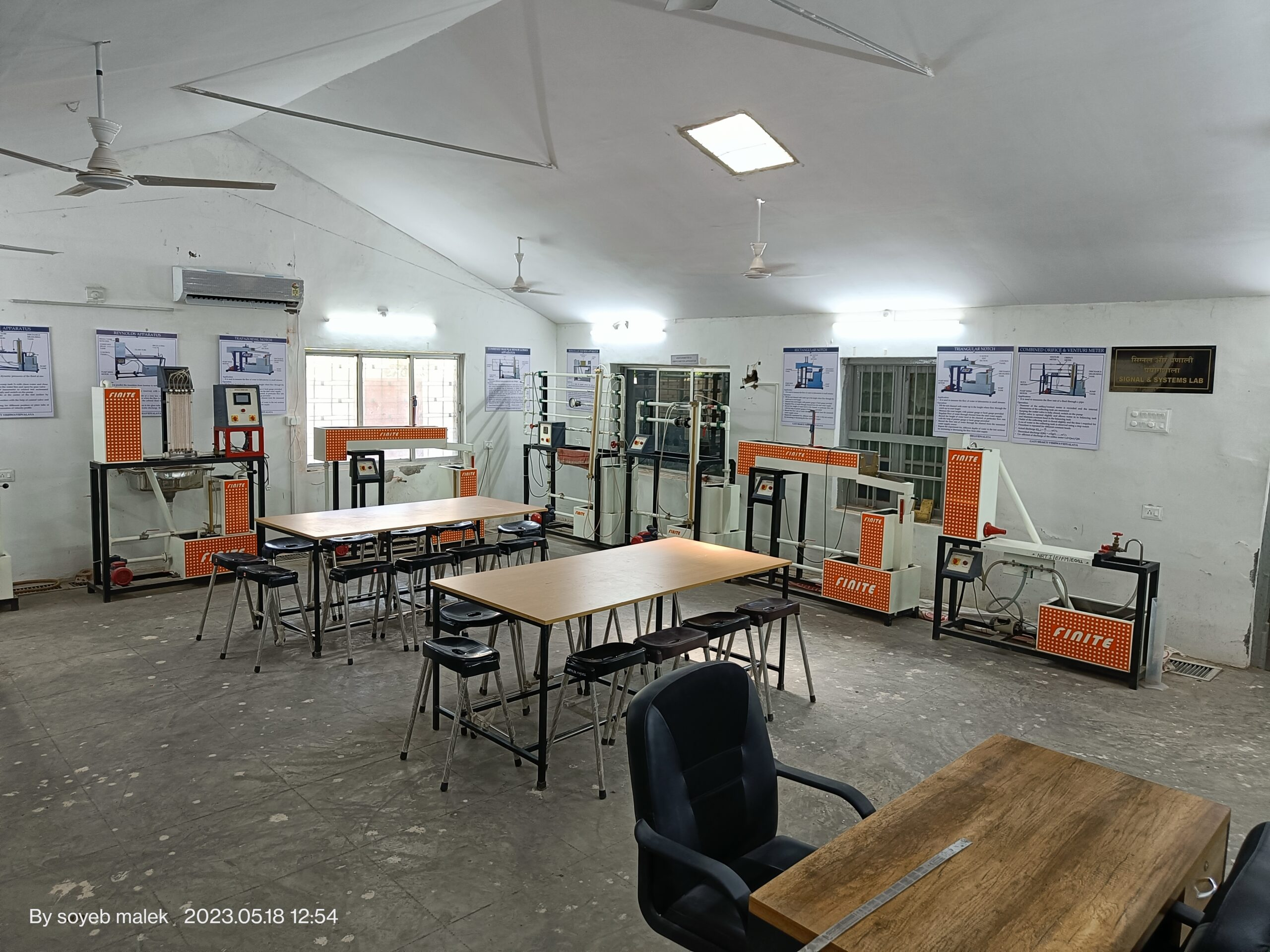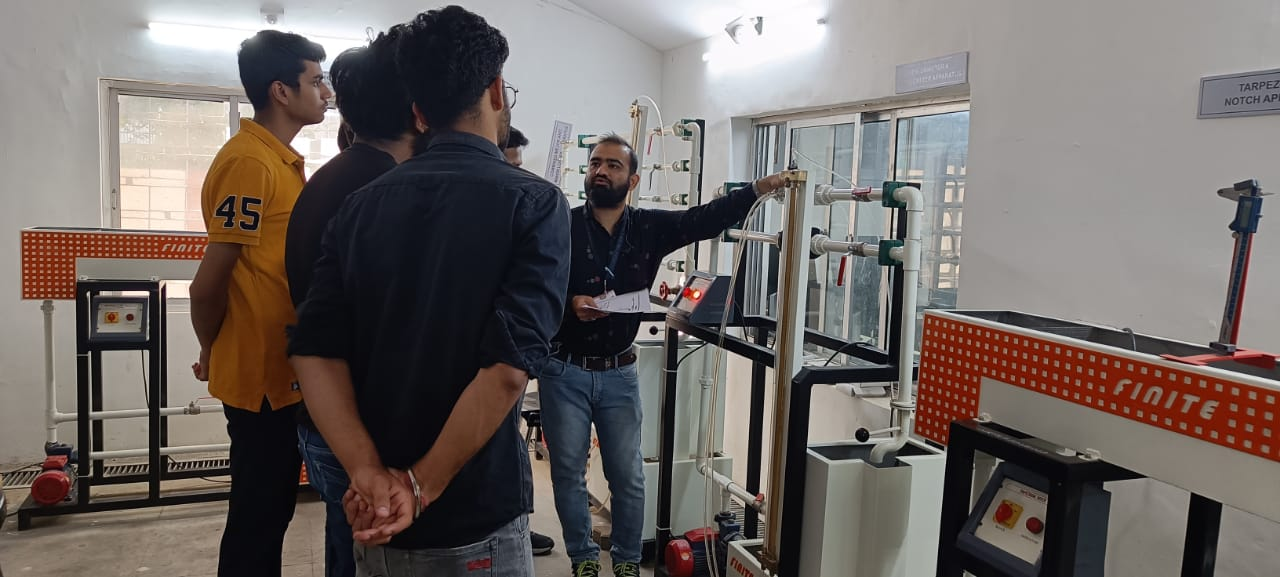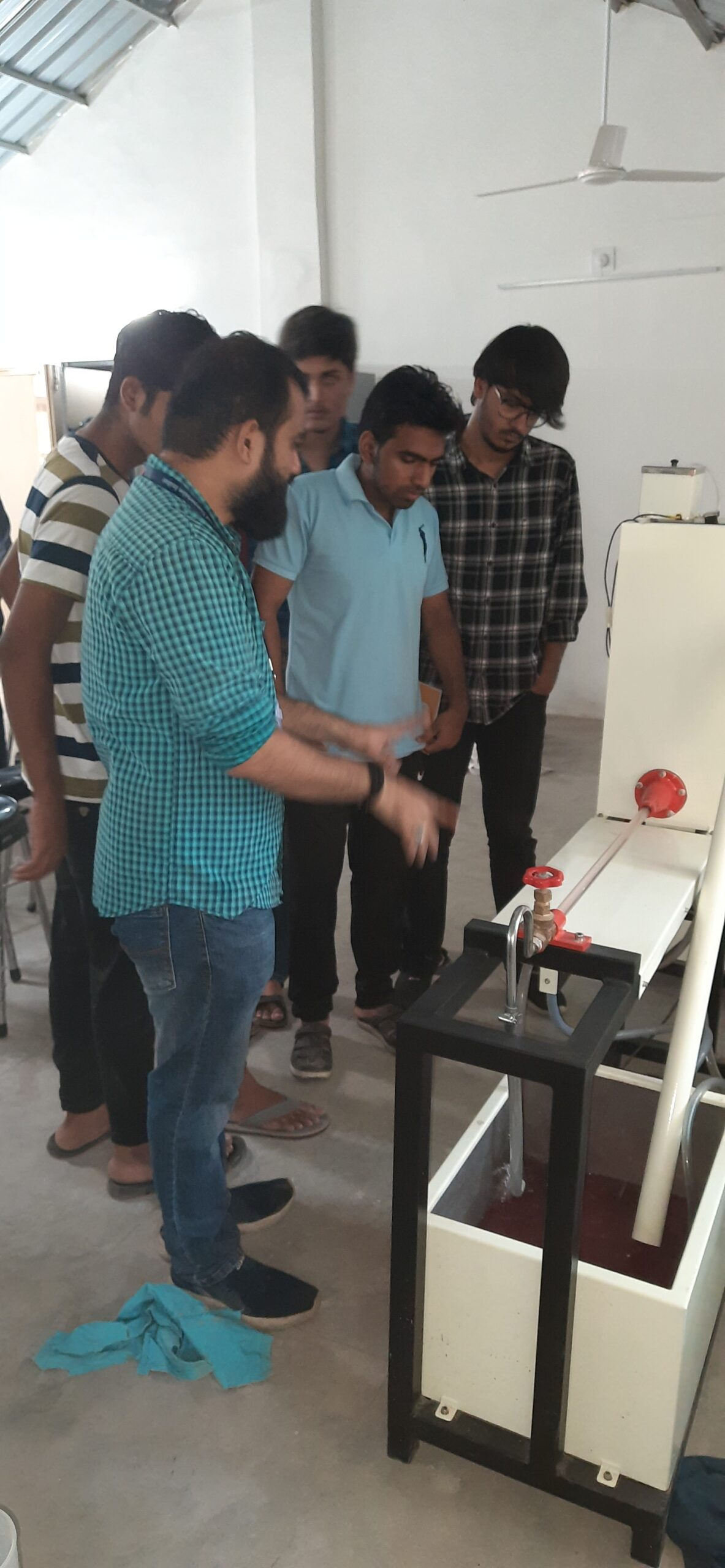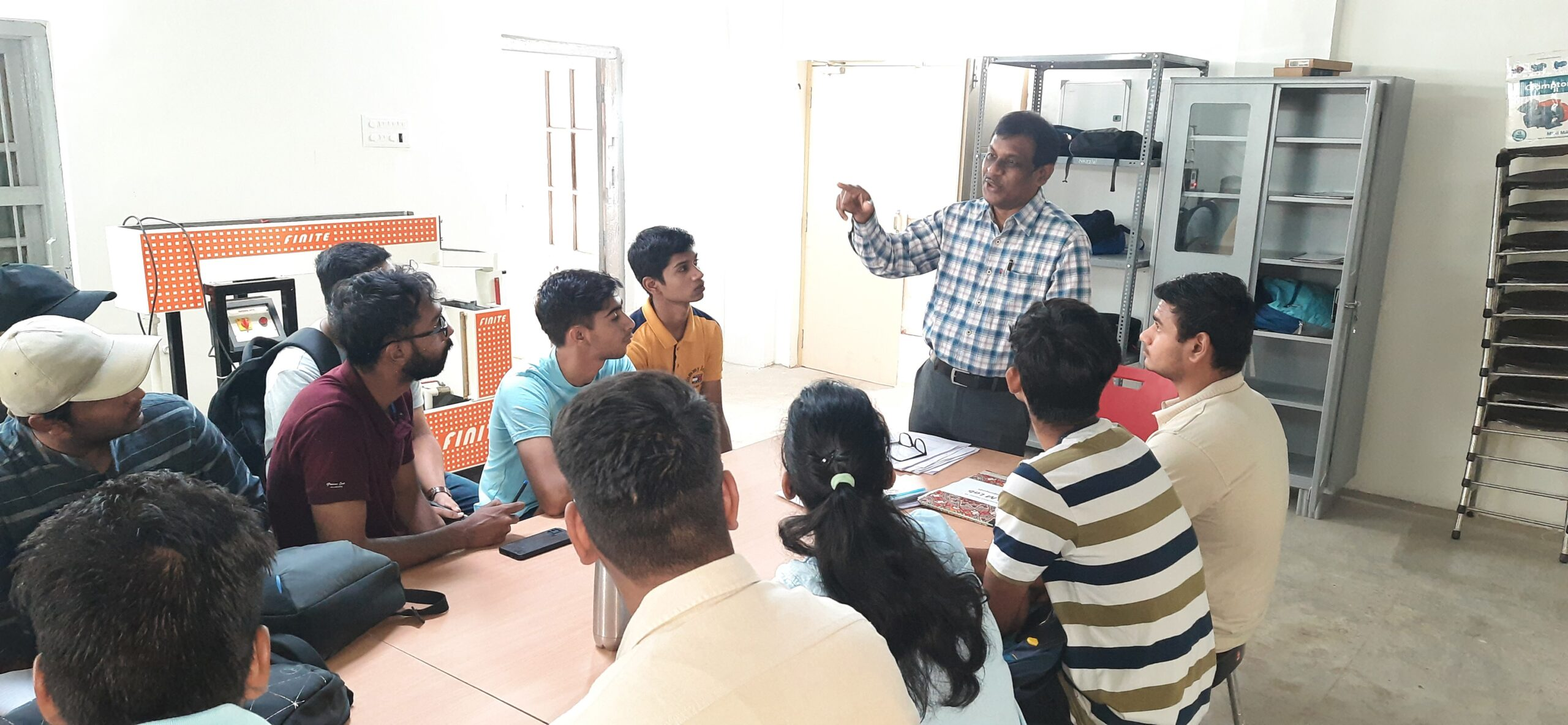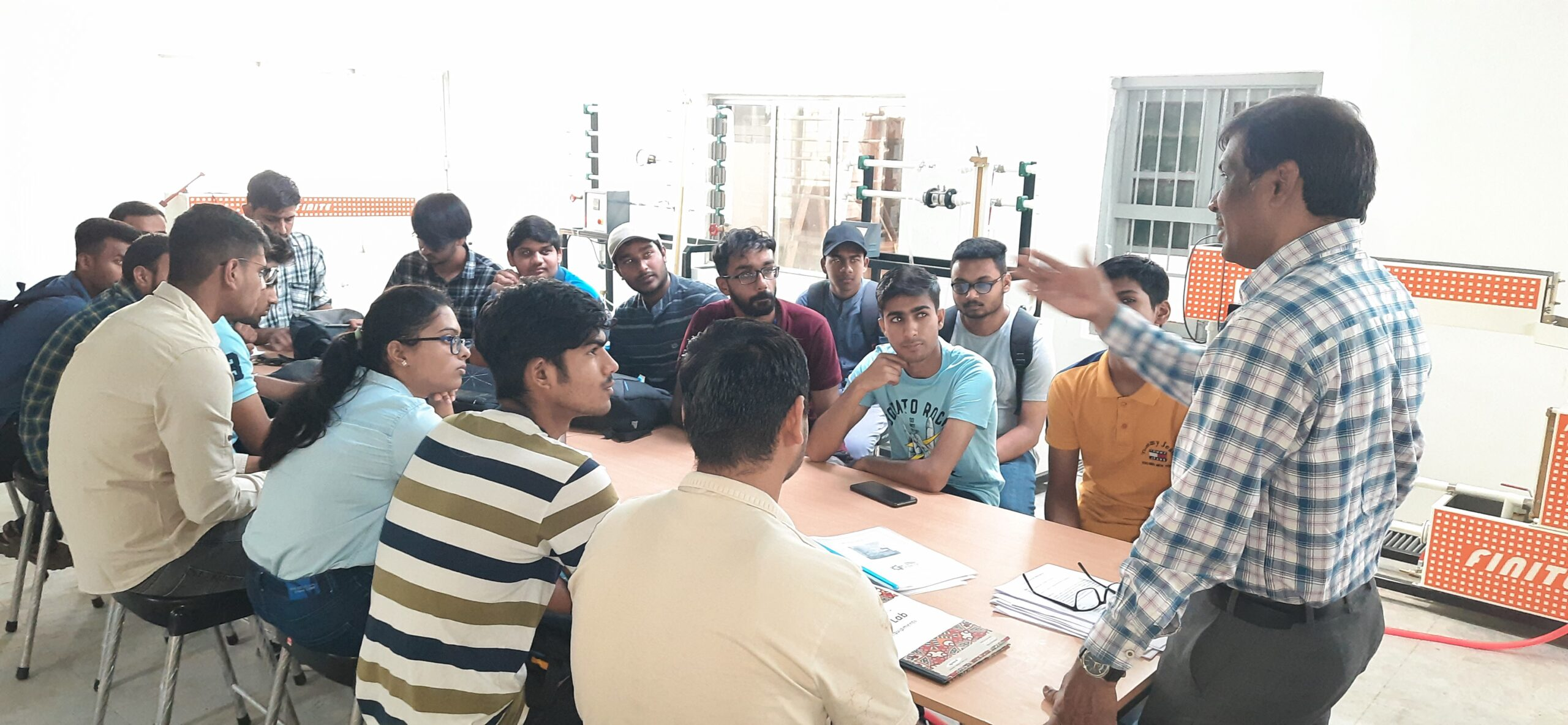demo

## FLUID MECHANICS LAB

The Fluid Mechanics laboratory is to examine the properties of fluids and to conduct experiments involving both incompressible and compressible flow. It is a course where flow behaviour, fluid forces and analysis tools are introduced. The goals of the experiments include determination of forces generated when fluid flow takes place over a solid object, applications of the control volume approach, demonstration of the momentum and energy equations, viscosity measurement and engineering correlations. Intricate flow phenomena such as separations and transition to turbulence are demonstrated. Experimental setups such as flow through a tube, flow over a flat plate, flow through venture meter and orifice are available to the students

LAB OBJECTIVES:

1. Enrich the concept of fluid mechanics and hydraulic machines.
2. Demonstrate the classical experiments in fluid mechanics and hydraulic machinery.
3. Correlate various flow measuring devices such as Venturimeter, orifice meter and notches etc.
4. Discuss the performance characteristics of turbines and pumps

### EXPERIMENTS:

1. Demonstration of Bernoulli’s Theorem.
2. Demonstration of Laminar and Turbulent Flow through Reynolds’s Apparatus.
3. Observation of rate of flow using rotameter and digital flow meter
4. Determination of flow rate using orifice meter
5. Determination of flow rate using venturi meter
6. Determination of the co-efficient of discharge of rectangular notch.
7. Determination of the co-efficient of discharge of V shaped notch.
8. Determination of the co-efficient of discharge of Trapezoidal notch.
9. Determination of friction factor in pipe flows.
10. Determination of loss of head of various pipe fittings.